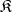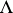# Free algebra

(diff) ← Older revision | Latest revision (diff) | Newer revision → (diff)
in a classof universal algebras
An algebrainwith a free generating system (or base), that is, with a setof generators such that every mapping ofinto any algebrafromcan be extended to a homomorphism ofinto(see Free algebraic system). Any non-empty class of algebras that is closed under subalgebras and direct products and that contains non-singleton algebras, has free algebras. In particular, free algebras always exist in non-trivial varieties and quasi-varieties of universal algebras (see Variety of universal algebras; Algebraic systems, quasi-variety of). A free algebra in the class of all algebras of a given signatureis called absolutely free. An algebraof signatureis a free algebra in some class of universal algebras of signatureif and only ifis intrinsically free, that is, if it has a generating setsuch that every mapping ofintocan be extended to an endomorphism of. If a free algebra has an infinite base, then all its bases have the same cardinality (see Free Abelian group; Free algebra over a ring; Free associative algebra; Free Boolean algebra; Free group; Free semi-group; Free lattice; Free groupoid; Free module; and also Free product). Clearly, every element of a free algebra with a basecan be written as a word over the alphabetin the signature of the class being considered. It is natural to ask: When are different words equal as elements of the free algebra? In certain cases the answer is almost trivial (semi-groups, rings, groups, associative algebras), while in others it is fairly complicated (Lie algebras, lattices, Boolean algebras), and sometimes it does not have a recursive solution (alternative rings).